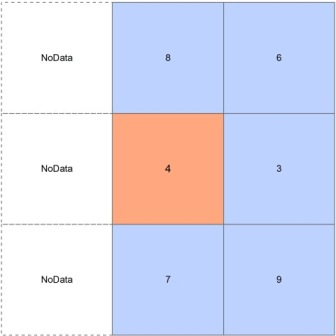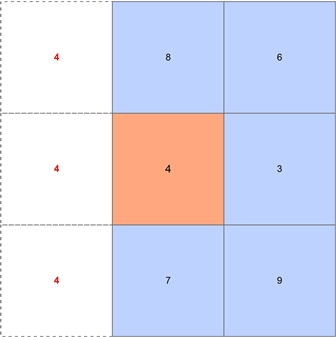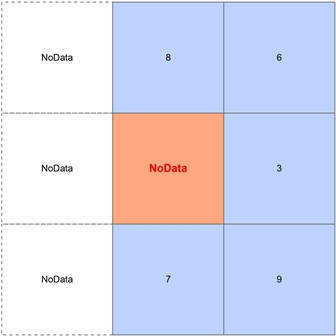English

# FAQ: How are pixels at the perimeter of a raster handled in the Slope tool versus the Slope function?

## Question

How are pixels at the perimeter of a raster handled in the Slope tool versus the Slope function?

The boundary raster cell values are calculated differently when using the Slope tool versus when applying a Slope function to an elevation raster or mosaic dataset. Therefore different results can be returned when running tools like Extract Values to Points to get slope values at the perimeter of a slope raster versus that of an elevation mosaic dataset with the Slope Function applied. Explanation below illustrates how boundary cells are handled differently in both cases.

The Slope tool (within the Spatial Analyst extension or 3D Analyst extension) requires an immediate neighborhood (3 x 3 window) to calculate the rate of maximum change in z-value for the center cell. If any neighborhood cells are NoData, they are assigned the value of the center cell, then the slope is computed. For instance, a perimeter cell with a value of 4, some cells within its 3 x 3 neighborhood are NoData, the Slope tool will assume them to be the same value as the center cell, which is 4 in this case, then calculates the slope value.

However, the Slope function in a mosaic dataset does not assume pixel values. If any neighborhood cells are NoData, the center cell will be NoData in the output slope raster.

Therefore, when running tools like Extract Values to Points on points located at these boundary pixels, a value of -9999 (NoData) may be received for slope values when the slope raster was created using the Slope Function, and instead get data values for the same boundary cells if the raster was created from the Slope tool.